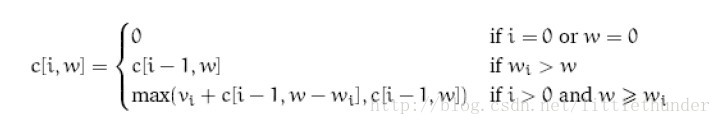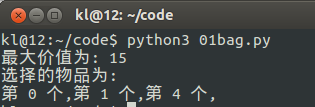•# Python基于动态规划算法解决01背包问题实例

Python基于动态规划算法解决01背包问题实例defbag(n,c,w,v):
res=[[-1forjinrange(c+1)]foriinrange(n+1)]
forjinrange(c+1):
res[j]=0
foriinrange(1,n+1):
forjinrange(1,c+1):
res[i][j]=res[i-1][j]
ifj>=w[i-1]andres[i][j]<res[i-1][j-w[i-1]]+v[i-1]:
res[i][j]=res[i-1][j-w[i-1]]+v[i-1]
returnres
defshow(n,c,w,res):
print('最大价值为:',res[n][c])
x=[Falseforiinrange(n)]
j=c
foriinrange(1,n+1):
ifres[i][j]>res[i-1][j]:
x[i-1]=True
j-=w[i-1]
print('选择的物品为:')
foriinrange(n):
ifx[i]:
print('第',i,'个,',end='')
print('')
if__name__=='__main__':
n=5
c=10
w=[2,2,6,5,4]
v=[6,3,5,4,6]
res=bag(n,c,w,v)
show(n,c,w,res)CDA考试指南
CDA数据分析员课程手册
CDA一级业务分析师课程手册
CDA二级建模分析师课程手册
CDA二级大数据分析师课程手册
CDA脱产就业班课程手册
CDA一级前导试听视频
CDA二级建模前导试听视频
CDA二级大数据前导试听视频
CDA招生简章及价格手册

OK,　确定提交

##